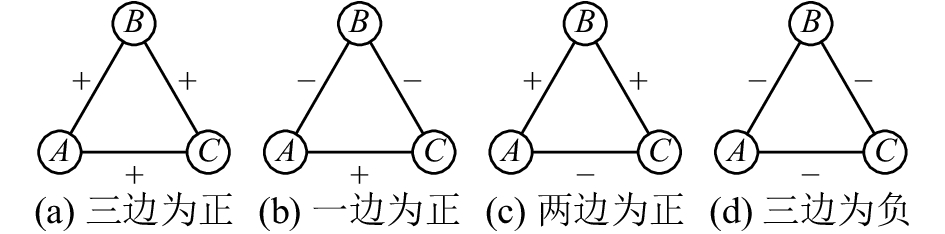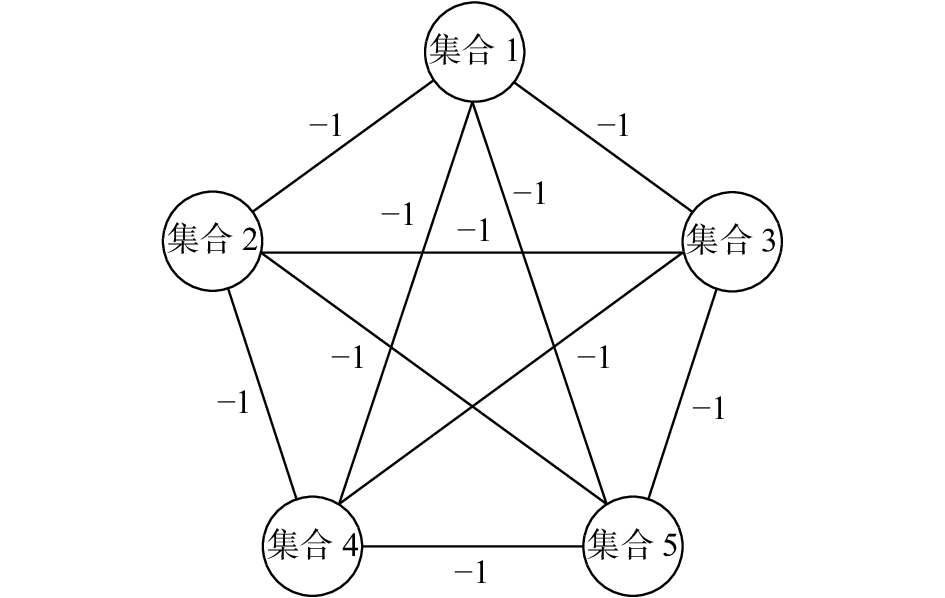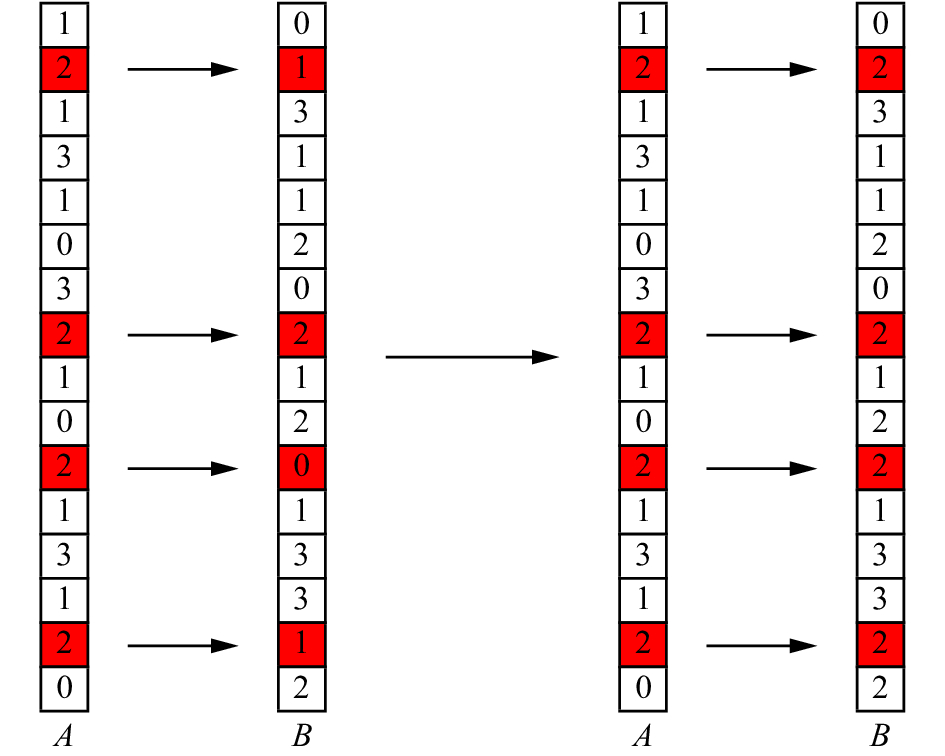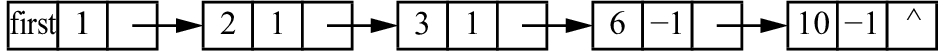﻿ WSB-EA进化算法的符号网络弱结构平衡分析
«上一篇文章快速检索 高级检索

 智能系统学报2018, Vol. 13Issue (5): 783-790  DOI: 10.11992/tis.2017060540

### 引用本文CHANG Xingong, ZHAO Yajuan. Weak structure balance analysis of signed network based on WSB-EA evolutionary algorithm[J]. CAAI Transactions on Intelligent Systems, 2018, 13(5): 783-790. DOI: 10.11992/tis.201706054.### 文章历史

WSB-EA进化算法的符号网络弱结构平衡分析

Weak structure balance analysis of signed network based on WSB-EA evolutionary algorithm
CHANG Xingong, ZHAO YajuanFaculty of Information Management, Shanxi University of Finance and Economics, Taiyuan 030006, China
Abstract: The weak structural balance (WSB) theory is suitable to solve the weak structure balance problem of most signed networks, and it is an NP-hard problem. Here a WSB evolutionary algorithm (WSB-EA) is proposed, which computes the global unbalanced degree of signed network based on evolutionary algorithm. In this method, the energy function of WSB theory is used as the fitness function. First, a heuristic method is used to initialize the population. After the tournament selection, single crossing, single point variation, and local search, the optimal solution is obtained after a finite number of iterations. The algorithm involves a storage of large signed network and incremental calculation. Through several experiments, the weak unbalanced degree of four small signed networks and two large signed networks are derived from WSB-EA algorithm. Compared with other algorithms, the WSB-EA algorithm can converge to the optimal solution faster and has a higher robustness.
Key words: signed network    evolutionary algorithm    NP-hard problem    structural balance theory    weak structural balance theory    single cross    local search    weak unbalanced degree

1 背景知识

1.1 弱结构平衡定理Download: 图 1 符号网络中4种三角形结构 Fig. 1 Four triangle signed network structuresDownload: 图 2 弱结构平衡网络 Fig. 2 Weak balance network structure
1.2 弱结构平衡定理能量函数

 $h\left( s \right) = \sum\limits_{ij} {\left( {1 - {J_{ij}}\delta \left( {{s_i},{s_j}} \right)} \right)} /2$ (1)

1.3 进化算法

2 WSB-EA算法描述 2.1 WSB-EA算法主要流程

1) ${\rm put}{ J}$ (); //存储邻接矩阵

2) Pop←Population(P, true); //初始化种群

3) a=0;

4) repeat

5) individual = Pop.getFittest();//计算得到

6) Pop←evolvePopulation(Pop, individual); //种群进化，选择、交叉、变异操作

7) a=a+1;

8) until a=M //达到最大进化次数时停止

2.2 编码

2.3 初始化

2.4 遗传操作

1) newPop.saveIndividua(0, individual); //精英保留

2) for( j=0; j< Pop.size();j++)

3) indiv1 =tournamentSelection(Pop); //选择父本1

4) indiv2 = tournamentSelection(Pop);

5) newIndiv=crossover(indiv1, indiv2); //交叉算法

6) newPop.saveIndividua ( j, newIndiv);

7) for( j=0; j< Pop.size();j++)

8) mutate(newPop.getIndividual( j)); //变异算法

2.4.1 选择操作

2.4.2 交叉操作Download: 图 3 单路交叉 Fig. 3 Single cross
2.4.3 变异操作

2.5 符号网络存储Download: 图 4 二维链表示例 Fig. 4 Two-dimension link structure
2.6 增量计算

 ${h_{\rm {new}}} = {h_{\rm {old}}} + \sum\limits_{{v_j} \in N\left( {{v_h}} \right)} {{J_{hj}}\left( {\delta \left( {{\rm {new}},{s_j}} \right) - \delta \left( {{\rm {old}},{s_j}} \right)} \right)}$

 $\begin{array}{c}{{\textbf{证明}}}\qquad\qquad h = \displaystyle\sum\limits_{{v_i},{v_j} \in E} {{J_{ij}}\delta \left( {{s_i},{s_j}} \right)} = \qquad\qquad\qquad \\ \displaystyle\sum\limits_{{v_h},{v_j} \in E} {{J_{hj}}\delta \left( {{s_h},{s_j}} \right)} + \sum\limits_{{v_i},{v_j} \in E \wedge i! = h} {{J_{ij}}\delta \left( {{s_i},{s_j}} \right)} = \\ \displaystyle\sum\limits_{{v_j} \in N\left( {{v_h}} \right)} {{J_{hj}}\delta \left( {{s_h},{s_j}} \right)} + \sum\limits_{{v_i},{v_j} \in E \wedge i! = h} {{J_{ij}}\delta \left( {{s_i},{s_j}} \right)} \end{array}$ (2)

 $\begin{array}{c}{h_{\rm {new}}} - {h_{\rm {old}}} =\\ \displaystyle \sum\limits_{{v_j} \in N\left( {{v_h}} \right)} {{J_{hj}}\delta \left( {{\rm {new}},{s_j}} \right)} - \sum\limits_{{v_j} \in E\left( {{v_h}} \right)} {{J_{hj}}\delta \left( {{\rm {old}},{s_j}} \right)} =\\ \displaystyle \sum\limits_{{v_j} \in N\left( {{v_h}} \right)} {{J_{hj}}\left( {\delta \left( {{\rm {new}},{s_j}} \right) - \delta \left( {{\rm {old}},{s_j}} \right)} \right)} \end{array}$

1) increaseFitness=0；

2) c =Data.node[id].first;

3) 遍历节点id的所有邻居节点；

4) if (正邻居)

5) if(getGene(c.data)==old&&getGene(c.data)!=new)

6) increaseFitness= increaseFitness+2；

7) else if(getGene(c.data)!=old&&getGene(c.data)== new)

8) increaseFitness= increaseFitness-2；

9) else if(负邻居)

10) if(getGene(c.data)==old&&getGene (c.data) !=new)

11) increaseFitness= increaseFitness-2；

12) else if(getGene(c.data)!=old&&getGene (c.data) == new)

13) increaseFitness= increaseFitness+2；

2.7 复杂性分析

3 实验结果与分析 3.1 参数设置表 1 参数设置 Tab.1 Parameter setting
3.2 小型符号网络实验结果

Gahuku-Gama部落网络：这一网络中有16个节点，代表16个部落。边数为59，代表部落之间的联盟和对抗。

Yeast网络：这是一个酵母菌的基因调控网络，该网络包含690个节点和1 080条边。由于这一网络的节点个数较多，在实验中将种群规模增加到500。表 2 对比实验结果1 Tab.2 Comparison results 1表 3 对比实验结果2 Tab.3 Comparison results 2表 4 4种小型符号网络的弱不平衡度实验结果 Tab.4 The experimental results of unbalanced degree of four small signed networks
3.3 大型符号网络实验结果

Epinions网络：Epinions网络是从Epinions获取的，Epinions网站是一般消费者评论网站，成立于1999年。游客可以对各种物品进行评价，也可以通过阅读各种物品的评价来帮助他们作出购买决策。该网络有131 828个节点，841 372条边。每条连边表示节点之间的信任关系。此数据集是有向网络，因此预处理数据时需将其改为无向网络，即将节点之间关系冲突的连边删除，例如节点1认可节点2评论，但节点2不认可节点1的评论，在预处理数据时要删除这样的连边。

Slashdot网络：Slashdot网络是从Slashdot网站获取的，Slashdot网站是一个资讯科技网站。它每天都会更新主页的新闻数次，网站使用者可以对公布在该站的新闻发表意见。该网络有81871个节点，545 671条边。预处理的方法与处理Epinions数据集方法一样。表 5 两种大型符号网络的弱不平衡度实验结果 Tab.5 The experimental results of unbalanced degree of two large signed network
4 结束语

  程苏琦, 沈华伟, 张国清, 等. 符号网络研究综述[J]. 软件学报, 2014, 25(1): 1-15. CHENG Suqi, SHEN Huawei, ZHANG Guoqing, et al. Survey of signed network research[J]. Journal of software, 2014, 25(1): 1-15. (0)  蓝梦微, 李翠平, 王绍卿, 等. 符号社会网络中正负关系预测算法研究综述[J]. 计算机研究与发展, 2015, 52(2): 410-422. LAN Mengwei, LI Cuiping, WANG Shaoqing, et al. Survey of sign prediction algorithms in signed social networks[J]. Journal of computer research and development, 2015, 52(2): 410-422. (0)  AMARAL L A N, SCALA A, BARTHELEMY M, et al. Classes of behavior of small-world networks[J]. arXiv: cond-mat/0001458, 2000. (0)  MOORE M. An international application of Heider’s balance theory[J]. European journal of social psychology, 1978, 8(3): 401-405. DOI:10.1002/(ISSN)1099-0992 (0)  PARISIEN C, ANDERSON C H, ELIASMITH C. Solving the problem of negative synaptic weights in cortical models[J]. Neural computation, 2008, 20(6): 1473-1494. DOI:10.1162/neco.2008.07-06-295 (0)  HEIDER F. Attitudes and cognitive organization[J]. The journal of psychology, 1946, 21(1): 107-112. DOI:10.1080/00223980.1946.9917275 (0)  LESKOVEC J, HUTTENLOCHER D, KLEINBERG J. Signed networks in social media[C]//Proceedings of the SIGCHI Conference on Human Factors in Computing Systems. Atlanta, USA, 2010: 1361–1370. (0)  孙一翔. 基于进化算法的符号网络结构平衡分析[D]. 西安: 西安电子科技大学, 2014: 25–36. SUN Yixiang. Structural balance analysis in signed networks based on evolutionary algorithm[D]. Xi'an: Xidian University, 2014: 25–36. (0)  CARTWRIGHT D, HARARY F. Structural balance: a generalization of Heider’s theory[J]. Psychological review, 1956, 63(5): 277-293. DOI:10.1037/h0046049 (0)  DAVIS J A. Clustering and structural balance in graphs[J]. Human relations, 1967, 20(2): 181-187. DOI:10.1177/001872676702000206 (0)  FOGEL D B. An introduction to simulated evolutionary optimization[J]. IEEE transactions on neural networks, 1994, 5(1): 3-14. DOI:10.1109/72.265956 (0)  FOGEL D B. Evolutionary algorithms in theory and practice[J]. Complexity, 1997, 2(4): 26-27. DOI:10.1002/(ISSN)1099-0526 (0)  廖美英, 郭荷清, 张勇军. 一种整数编码的改进遗传算法[J]. 计算机工程与应用, 2003, 39(1): 103-105, 120. LIAO Meiying, GUO Heqing, ZHANG Yongjun. An ameliorative integer coded genetic algorithm[J]. Computer engineering and applications, 2003, 39(1): 103-105, 120. DOI:10.3321/j.issn:1002-8331.2003.01.034 (0)  邓琨, 张健沛, 杨静. 利用改进遗传算法进行复杂网络社团发现[J]. 哈尔滨工程大学学报, 2013, 34(11): 1438-1444. DENG Kun, ZHANG Jianpei, YANG Jing. Community detection in complex networks using an improved genetic algorithm[J]. Journal of Harbin engineering university, 2013, 34(11): 1438-1444. (0)  YANG Bo, CHEUNG W, LIU Jiming. Community mining from signed social networks[J]. IEEE transactions on knowledge and data engineering, 2007, 19(10): 1333-1348. DOI:10.1109/TKDE.2007.1061 (0)  KROPIVNIK S, MRVAR A. An analysis of the slovene parliamentary parties network[M]//FERLIGOJ A, KRAMBERGER A. Developments in Statistics and Methodology. FDV, Ljubljana: Metodološki Zvezki, 1996. (0)  READ K E. Cultures of the central highlands, new guinea[J]. Southwestern journal of anthropology, 1954, 10(1): 1-43. DOI:10.1086/soutjanth.10.1.3629074 (0)  MILO R, SHEN-ORR S, ITZKOVITZ S, et al. Network motifs: simple building blocks of complex networks[J]. Science, 2002, 298(5594): 824-827. DOI:10.1126/science.298.5594.824 (0)  FACCHETTI G, IACONO G, ALTAFINI C. Computing global structural balance in large-scale signed social networks[J]. Proceedings of the national academy of sciences of the United States of America, 2011, 108(52): 20953-20958. DOI:10.1073/pnas.1109521108 (0)